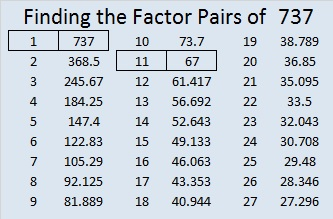# 737 and Level 6

• 737 is a composite number.
• Prime factorization: 737 = 11 x 67
• The exponents in the prime factorization are 1 and 1. Adding one to each and multiplying we get (1 + 1)(1 + 1) = 2 x 2 = 4. Therefore 737 has exactly 4 factors.
• Factors of 737: 1, 11, 67, 737
• Factor pairs: 737 = 1 x 737 or 11 x 67
• 737 has no square factors that allow its square root to be simplified. √737 ≈ 27.14774392.Here is a challenging level 6 puzzle. Some possible steps to solve it are in a table at the end of this post.Print the puzzles or type the solution on this excel file: 10 Factors 2016-01-04

———————————————————

The Boeing 737 is the best selling commercial airline jet. It has been produced continuously since 1967. If you’ve ever flown Southwest Airlines, Ryanair, United Airlines, or American Airlines, chances are the 737 took you to your destination.

The next time you fly in a 737, use the following number facts to impress your seatmates. They will be so glad they sat next to you: 😉

7 – 3 + 7 = 11 so 737 is divisible by 11.

737 can be written as the sum of consecutive numbers three ways:

• 368 + 369 = 737; that’s 2 consecutive numbers.
• 62 + 63 + 64 + 65 + 66 + 67 + 68 + 69 + 70 + 71 + 72 = 737; that’s 11 consecutive numbers.
• 23 + 24 + 25 + 26 + 27 + 28 + 29 + 30 + 31 + 32 + 33 + 34 + 35 + 36 + 37 + 38 + 39 + 40 + 41 + 42 + 43 + 44 = 737; that’s 22 consecutive numbers.

737 is obviously a palindrome, but base 10 is not the only base that can make that claim:

• 737 base 10; note that 7(100) + 3(10) + 7(1) = 737.
• 515 BASE 12; note that 5(144) + 1(12) + 5(1) = 737.
• 191 BASE 23; note that 1(529) + 9(23) + 1(1) = 737.

———————————————————